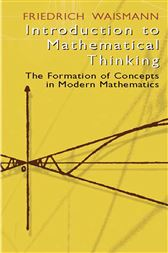Introduction to Mathematical Thinking

Series: Dover Books on MathematicsUS\$14.95
ISBNs
• 9780486428048
• 9780486167428

This enlightening survey of mathematical concept formation holds a natural appeal to philosophically minded readers, and no formal training in mathematics is necessary to appreciate its clear exposition of mathematic fundamentals. Rather than a system of theorems with completely developed proofs or examples of applications, readers will encounter a coherent presentation of mathematical ideas that begins with the natural numbers and basic laws of arithmetic and progresses to the problems of the real-number continuum and concepts of the calculus.
Contents include examinations of the various types of numbers and a criticism of the extension of numbers; arithmetic, geometry, and the rigorous construction of the theory of integers; the rational numbers, the foundation of the arithmetic of natural numbers, and the rigorous construction of elementary arithmetic. Advanced topics encompass the principle of complete induction; the limit and point of accumulation; operating with sequences and differential quotient; remarkable curves; real numbers and ultrareal numbers; and complex and hypercomplex numbers.
In issues of mathematical philosophy, the author explores basic theoretical differences that have been a source of debate among the most prominent scholars and on which contemporary mathematicians remain divided. "With exceptional clarity, but with no evasion of essential ideas, the author outlines the fundamental structure of mathematics." — Carl B. Boyer, Brooklyn College. 27 figures. Index.

ISBNs
• 9780486428048
• 9780486167428
ISBNs
• 9780486428048
• 9780486167428